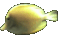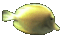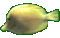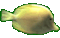Math Help - Name a Parabola Game - Use Y-intercept of Graph - Tips:

- A ball thrown upwards in still air follows an approximate parabolic arc.
- The curve y=-4x2+3 is a parabola that opens downwards and has a y-intercept of 3.
- The curve y=4x2+5 is a parabola that opens upwards and intersects the y-axis at 5.

- The second degree (or quadratic) relation y=ax2+b represents a set of ordered pairs (x,y)
which form a parabolic shape that passes through the point (0,b).
When 'a' is positive, the parabola opens upwards. When 'a' is negative, the parabola opens downwards.
Increasing the size of 'a', narrows the width of the mouth of the parabola.
When 'a' is zero, the parabola y=ax2+b reduces to the horizontal line y=b.

- Your Game Score is reduced by the number of fish hits.

- To slow the game speed repeat tap/click on the word Slider.
- To increase the game speed repeat tap/click on the word Math.
- Speed can also be adjusted with a keyboard's - and + keys.

- Refresh/Reload the web page to restart the game.